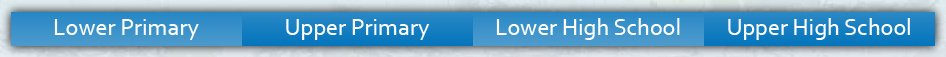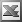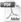Lower High School Enter keywords to search by topic or worksheet description

Worksheets in this category provide students with practice in factorising and solving quadratic and higher order equations.

Worksheet Name Files
Solving Quadratic Equations where a=1Solving Quadratic Equations where a>1Solving Quadratic Equations by Completing the SquareSolving Simple Quadratic EquationsSolving Quadratics by Formula